Courses

# Test: Symmetry - 3

## 20 Questions MCQ Test Mathematics (Maths) Class 7 | Test: Symmetry - 3

Description
This mock test of Test: Symmetry - 3 for Class 7 helps you for every Class 7 entrance exam. This contains 20 Multiple Choice Questions for Class 7 Test: Symmetry - 3 (mcq) to study with solutions a complete question bank. The solved questions answers in this Test: Symmetry - 3 quiz give you a good mix of easy questions and tough questions. Class 7 students definitely take this Test: Symmetry - 3 exercise for a better result in the exam. You can find other Test: Symmetry - 3 extra questions, long questions & short questions for Class 7 on EduRev as well by searching above.
QUESTION: 1

### Which of the following alphabets has a vertical line of symmetry?

Solution:

Recall definition of line symmetry.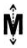QUESTION: 2

### Which of the following alphabets has a horizontal line of symmetry?

Solution:

All of the letters have horizontal line of symmetry since it cut them in equal parts if we draw a line in the middle of each of the letters.

QUESTION: 3

### Which of the following alphabets has no line of symmetry?

Solution:

P has no line of symmetry.

QUESTION: 4

Which of the following alphabets has many lines of symmetry?

Solution:

O has many lines of symmetry.

QUESTION: 5

Which of the following triangles has no line of symmetry?

Solution:

A scalene triangle has no line of symmetry.

QUESTION: 6

What is the mirror image of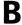in a horizontal mirror?

Solution:

Since horizontal mirror is mirror along with the x-axis , so B will be seen as B

QUESTION: 7

Which of the following figures are symmetrical with respect to exactly two lines?

Solution:

Only the third figure has horizontal and vertical symmetry. So it has 2 lines of symmetry.

QUESTION: 8

A Which of the following figures has rotational symmetry of order more than 1?

Solution:

Rotational Symmetry is how many times a shape fits into itself when rotated.So when a circle is rotated by 90 degree it fits into its original space. So its order is 4 since it fits into itself 4 times. An equilateral triangle when rotated by 120 degrees fits into itself. So its order is 3. The 3rd shape rotates the same as the circle. So its order is 4.

QUESTION: 9

A What is the order of rotational symmetry for the given figure?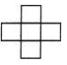Solution:
QUESTION: 10

What is the order of rotational symmetry for the given figure?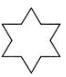Solution:
QUESTION: 11

Which of the following figures has only one line of symmetry?

Solution:

The second figure has only the vertical line of symmetry. So it has only 1 line of symmetry.

QUESTION: 12

Identify the number of lines of symmetry for the figure given.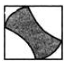Solution:

There are only two possibilities i.e., diagonal lines of symmetry. So there are two lines of symmetry.

QUESTION: 13

In the given figure, the dotted line is the line of symmetry. Which figure is formed if the given figure is reflected in the dotted line?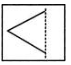Solution: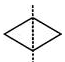The figure formed is a rhombus.

QUESTION: 14

What is the other name for a line of symmetry of a circle?

Solution:

A diameter divides the circle into 2 equal parts. So, it can be considered as a line of symmetry.

QUESTION: 15

A In A XYZ, XY = XZ and XM⊥YZand ZP⊥XY. About which of the following is the triangle symmetrical?

Solution: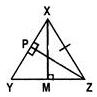Since XY=XZ and XM⊥YZ,

∠YXM=∠ZXM. So, the triangle is symmetrical about XM as it bisects the apex angle.

QUESTION: 16

What is the order of rotational symmetry of the English alphabet Z?

Solution:
QUESTION: 17

Which of these quadrilaterals have both line and rotational symmetries of order more than 3?

Solution:
QUESTION: 18

Which of these letters has only rotational symmetry?

Solution:
QUESTION: 19

A square has a rotational symmetry of order 4 about its centre. What is the angle of rotation?

Solution:
QUESTION: 20

What is the order of rotational symmetry of the figure given?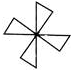Solution: